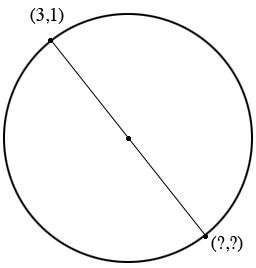SEARCH HOMEMath Central Quandaries & QueriesQuestion from Apple: One end of the the diameter of the circle with equation x^2+y^2-4x+4y=2 is (3,1). Find the coordinates of the other end of the diameter.Hi,

You have a circle and one point on it, (3,1). You want to construct the diagonal from (3,1) and find where this diagonal meets the circle.A diagonal must pass through the center of the circle, so It would be helpful to know the coordinates of the center. You can do that by using the technique I described in a response to a question from Ariana.

PennyMath Central is supported by the University of Regina and The Pacific Institute for the Mathematical Sciences.